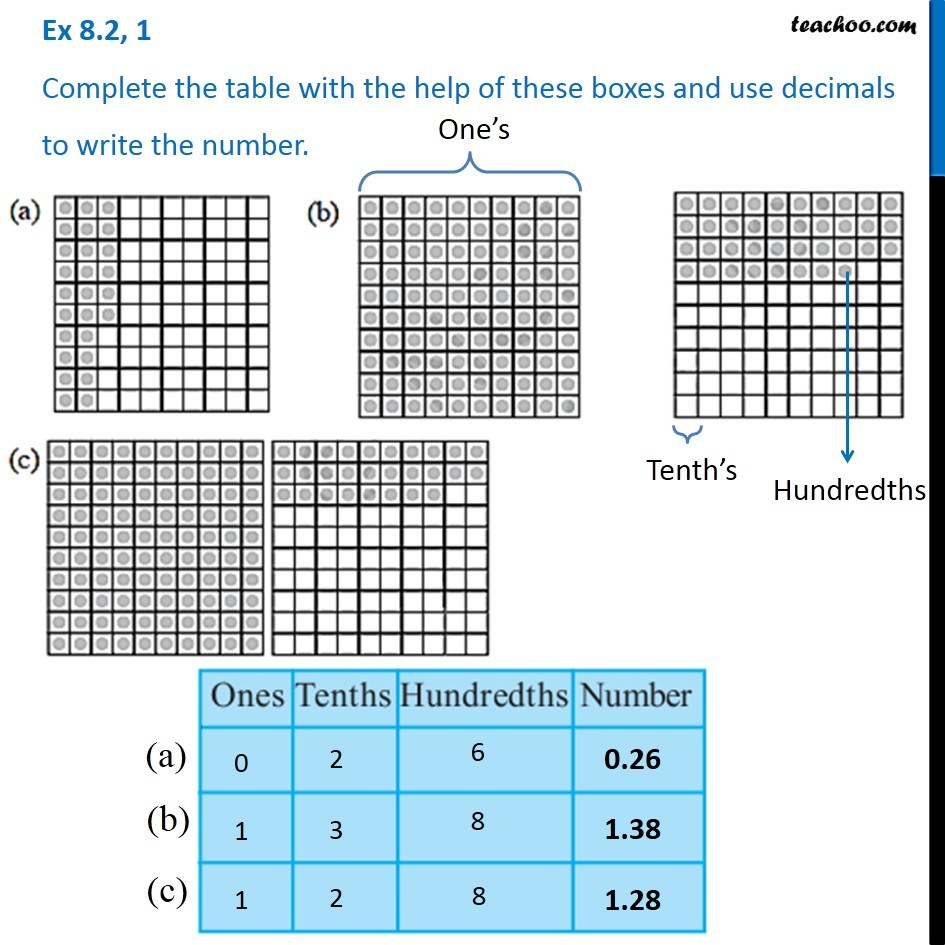Ex 8.2

Chapter 8 Class 6 Decimals
Serial order wiseGet live Maths 1-on-1 Classs - Class 6 to 12

### Transcript

Ex 8.2, 1 - Chapter 8 Class 6 NCERT Complete the table with the help of these boxes and use decimals to write the number. In the figure, one dot is Hundredths Ten dots are Tenths and 100 dots are Hundredths For (a) Ones (1) = 0 Tenths (1/10) = 2 Hundredths (1/100) = 6 Number is 0.26 For (b) Ones (1) = 1 Tenths (1/10) = 3 Hundredths (1/100) = 8 Number is 1.38 For (c) Ones (1) = 1 Tenths (1/10) = 2 Hundredths (1/100) = 8 Number is 1.28